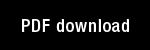An Ordering Algorithm for Pattern Presentation in Fuzzy ARTMAP That Tends to Improve Generalization Performance

Author(s): Bebis, G. | Dagher, I. | Georgiopoulos, M. | Heileman, G.L. |

Year: 1999

Citation: IEEE Transactions on Neural Networks, Vol. 10, No. 4, 768-778.

Abstract: In this paper we introduce a procedure, based on the max?min clustering method, that identifies a fixed order of training pattern presentation for fuzzy adaptive resonance theory mapping (ARTMAP). This procedure is referred to as the ordering algorithm, and the combination of this procedure with fuzzy ARTMAP is referred to as ordered fuzzy ARTMAP. Experimental results demonstrate that ordered fuzzy ARTMAP exhibits a generalization performance that is better than the average generalization performance of fuzzy ARTMAP, and in certain cases as good as, or better than the best fuzzy ARTMAP generalization performance. We also calculate the number of operations required by the ordering algorithm and compare it to the number of operations required by the training phase of fuzzy ARTMAP. We show that, under mild assumptions, the number of operations required by the ordering algorithm is a fraction of the number of operations required by fuzzy ARTMAP.

Topics: Machine Learning, Models: Fuzzy ARTMAP, Modified ART,